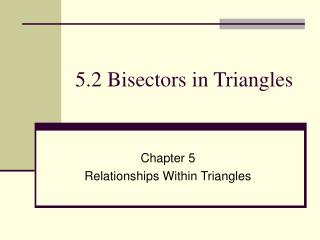# 5.2 Bisectors in Triangles - PowerPoint PPT PresentationDownload Presentation5.2 Bisectors in Triangles

5.2 Bisectors in Triangles
Download Presentation## 5.2 Bisectors in Triangles

- - - - - - - - - - - - - - - - - - - - - - - - - - - E N D - - - - - - - - - - - - - - - - - - - - - - - - - - -
##### Presentation Transcript

1. 5.2 Bisectors in Triangles Chapter 5 Relationships Within Triangles

2. 5.2 Bisectors in Triangles • Theorem 5-2 Perpendicular Bisector Theorem If a point is on the perpendicular bisector of a segment, then it is equidistant from the endpoints of the segment. • Theorem 5-3 Converse of the Perpendicular Bisector Theorem If a point is equidistant from the endpoints of a segment, then it is on the perpendicular bisector of the segment.

3. 5.2 Bisectors in Triangles C A D B AC = BC, so C is equidistant from the endpoints, and is on the perpendicular bisector

4. 5.2 Bisectors in Triangles • Theorem 5-4 Angle Bisector Theorem If a point is on the bisector of an angle, then the point is equidistant from the sides of the angle. • Theorem 5-5 Converse of the Angles Bisector Theorem If a point in the interior of an angle is equidistant from the sides of the angle, then the point is on the angle bisector

5. 5.2 Bisectors in Triangles • Point D is equidistant from the sides of the angle. • Distance is always perpendicular. C D A B

6. 5.2 Bisectors in Triangles • Find the value of x, then find FD and FB. C B D 5x 2x + 24 F

7. Practice • pg 252 12-26